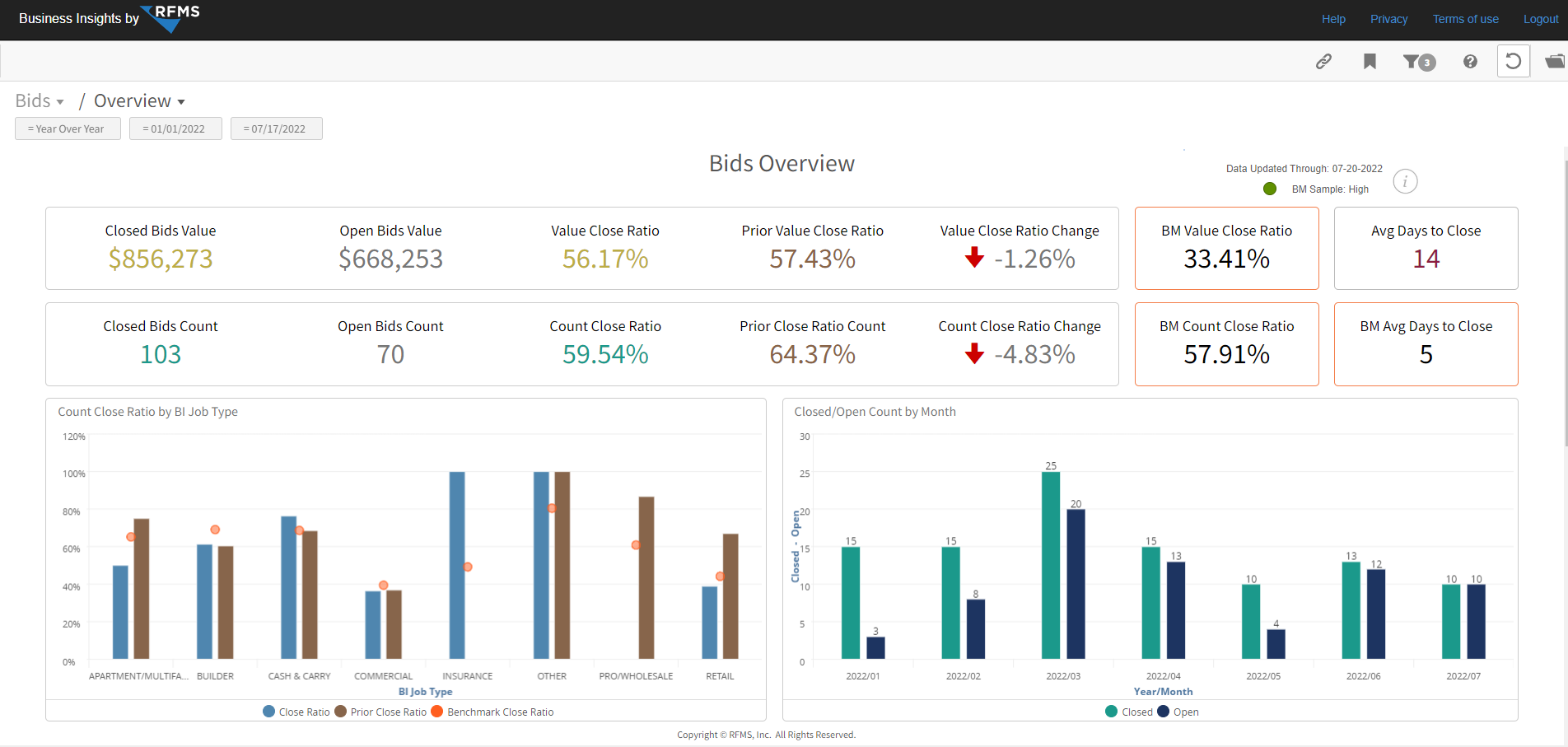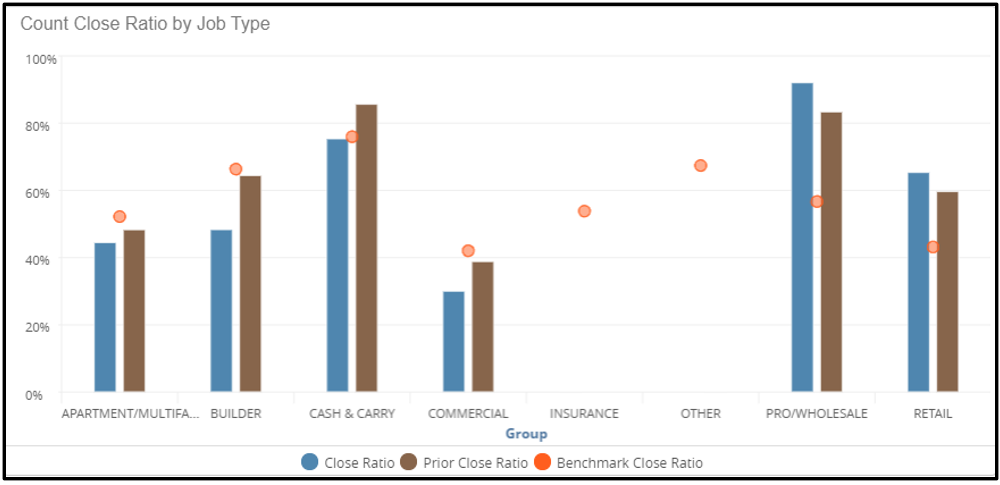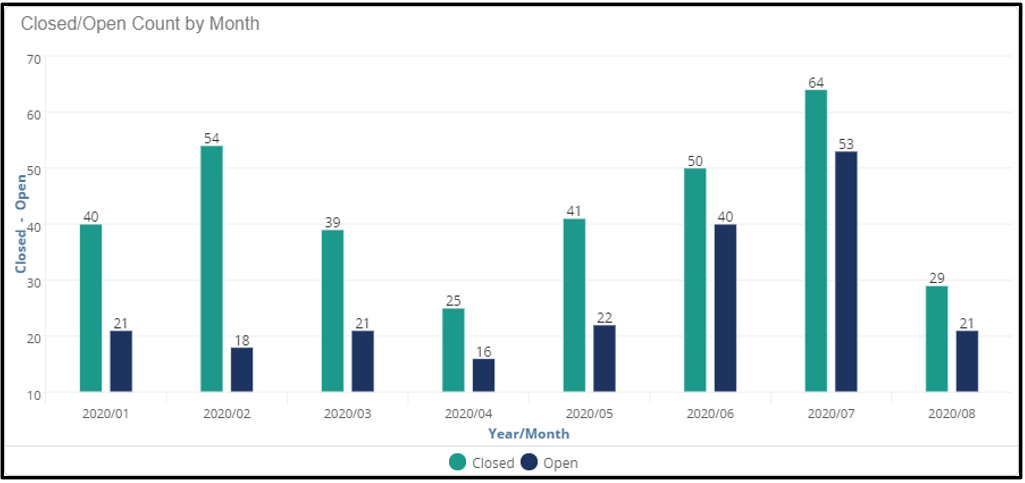# Bids Overview

This dashboard provides an overview of open and closed bids.Key Performance Indicator's (KPI's)

Closed Bids Value: The dollar value of closed (exported) bids for the active filters.

Open Bids Value: The dollar value of all open bids for the active filters.

Value Close Ratio: The percentage of existing bids that are closed for the selected filters. This is calculated by dividing Closed Bid Value by Total Bid Value.

Prior Value Close Ratio: The percentage of existing bids that are closed for the prior period of the selected date range (Year over Year or Trailing Months) for active filters. This value is calculated by dividing prior period Closed Bid Value by prior period Total Bid Value.

Value Close Ratio Change: The comparison of the prior period close ratio to the current close ratio. This is calculated by subtracting the Prior Value Close Ratio from the Value Close Ratio.

BM Value Close Ratio: The percentage of existing bids that are closed for the active filters for all qualified participating dealers. This is calculated by dividing all the Closed Bid Values by all the Total Bid Values.

Avg Days to Close: The average number of days to close bids.

Closed Bid Count: The number of closed bids.

Open Bids Count: The number open bids.

Count Close Ratio: The percentage of closed bids. This is calculated by dividing the number of closed bids by the total number of bids.

Prior Close Ratio Count: The percentage of closed bids. This is calculated by dividing the number of closed bids by the total number of bids for the prior period and selected filters.

Count Close Ratio Change: The difference between count close ratio and the prior close ratio count.

BM Count Close Ratio: The percentage of total number of closed bids divided by the total number of bids for qualified participating dealers for the active filters.

BM Avg Days to Close: Average number of days to close bids for all qualified participating dealers.

Charts

Count Close Ratio By Job Type: Count close ratio for each Job Type is represented by a blue (current period) column, brown (prior period) column and orange(benchmark) Indicator dot. Hovering over the visuals will display the Count close ratio as a percentage.

Note: For Job Type Mappings see the My Job Types chart under the Profile Collection.Closed/Open Count by Month: The closed bids are displayed in green and the open bids are displayed in dark blue by month for the time displayed in the chart legend. The time frame can be changed by modifying the date in the active filters.